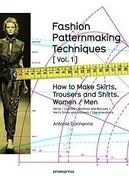# Basic Trousers With Pleats

Extract from Fashion Patternmaking Techniques. [ Vol. 1 ] • By Antonio Donnanno • Published by Promopress

Cost
\$ \$ \$ \$ \$
Difficulty
• • • • •
Time
2h00

Fashion Patternmaking Techniques
Measurements: - Hip circumf. 92+2-4 cm ease.
- Waist circumference 68+1-2 cm ease.
- Hip length 20 cm. - Body rise 24 cm.
- Trouser length 105 cm

Posted by Promopress Published See Promopress's 3 projects » © 2020 Antonio Donnanno / Promopress · Reproduced with permission.
•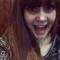GabriELLE favorited Basic Trousers With Pleats 14 Oct 05:46
•18 Mar 16:48
•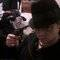Jac D. favorited Basic Trousers With Pleats 27 Apr 05:05
•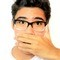12 Apr 02:55
•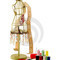08 Feb 01:57
•Suzi T. favorited Basic Trousers With Pleats 06 Feb 12:13
•Lee C. favorited Basic Trousers With Pleats 04 Feb 07:38
•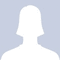kitblu favorited Basic Trousers With Pleats 01 Feb 23:25
•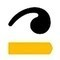Promopress published her project Basic Trousers With Pleats 12 Jan 12:50

#### You Will Need

•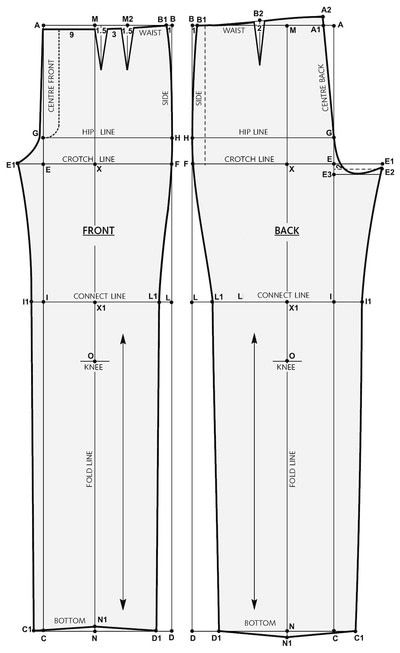Step 1

Front
- Draw a rectangle A-B-C-D, with A-B equal
to ¼ Hip circumference (e.g.: 92:4 = 2 cm)
and A-C equal to total trouser length
(e.g.: 105 cm).
- A-E is the body rise measurement
(24 cm) - B-F is the same as A-E.
- E-E1 = 1/16 the Hip circumf. minus 1.5
cm (e.g.: 92:16 = 5.7 - 1.5 = 4.2 cm).
- A-G is the Hip length measurement
(20 cm).
- Draw G-H (Hip line).
- E-I is the same as A-E.
- Draw I-L.
- E1-X = half of E1-F
(e.g.: 23+4.2 = 27.2:2 = 13.6 cm)
- Draw M-N passing through X and
X1, and write PLEAT LINE and GRAIN.
- M-O = Knee height (e.g.: 60 cm).
- M-M2 = 7 cm.
- Draw the darts with the length and
width required.
- B-B1 = 1 cm.
- X1-L1 = 12 cm.
- X1-I1 like X1-L1 (THIGH).
- N-N1 = 1 cm.
- N-C1 = 11 cm (or as desired).
- N-D1 like N-C1.
- Draw C1-N1-D1 (BOTTOM).
- Draw E1-G-A1 with a curved line.
- Draw E1-I1-C1 shaping it nicely.
- Draw B1-A1 shaping it nicely and write
WAIST.
- Draw B1-H-F-L1-D1, shaped nicely.

• Step 2

Back
- Draw a rectangle A-B-C-D, with:
- A-B equal to ¼ Hip circumference + 2 cm
(e.g.: 92:4 = 23+2 = 25 cm) and A-C equal to total
trouser length (e.g.: 105 cm).
- A-E is the body rise measurement (e.g.: 24 cm).
- B-F is the same as A-E.
- E-E1 1/16 Hip circumference + 3 cm.
(e.g.: 92:16 = 5.7+3 = 8.7 cm).
- E1-E2 = 1 cm.
- E-E3 = 2 cm.
- A-G is the Hip length measurement (e.g.: 20 cm).
- Draw G-H (Hip line).
- E-I is the same as A-E.
- Draw I-L.
- E1-X = half of E1-F (e.g.: cm 26+9 = 35:2 = 17.5).
- Draw M-N passing through X and write PLEAT LINE
and GRAIN.
- M-O Knee height. (e.g.: cm 60).
- A-A1 = 3.5 cm.
- A1- A2 = 1-3.5 cm.
- B-B1 = 1 cm (check waist curves).
- B1-B2 discard = ½ B1- A2.
- Draw the darts with desired length and width.
- X1-L1 = 13 cm.
- X1-I1 like X1- L1 (THIGH).
- N-n1 CM 1.
- N-C1 12 cm (or as desired).
- N-D1 like N-C1.
- Draw C1-N1-D1 (BOTTOM).
- Draw E2-G-A2 with a curved line.
- Draw E2-I1-C1 shaping it nicely.
- Draw B1- A2 shaping it nicely and write WAIST.
- Draw B1-H-F-L1-D1 shaped nicely.